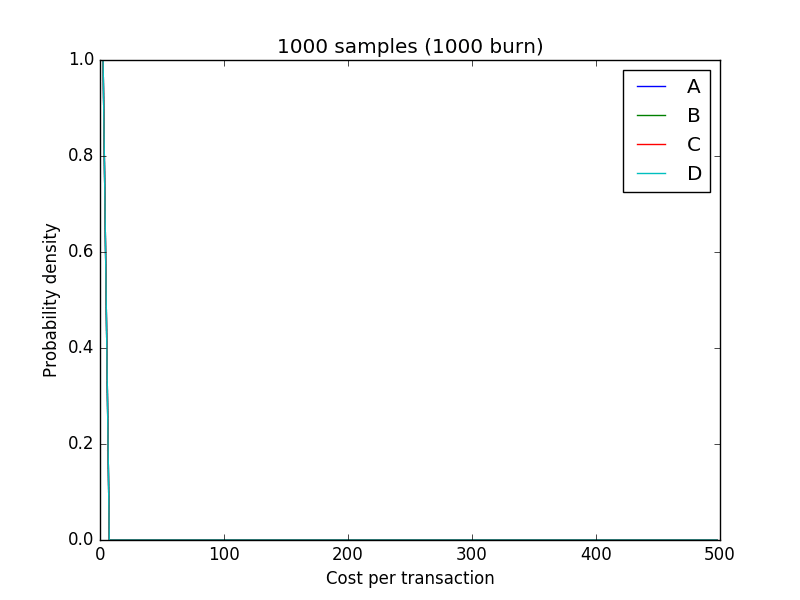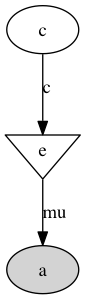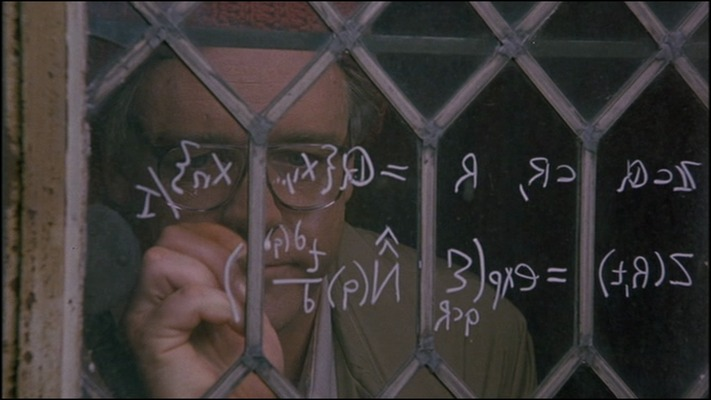# MCMC for marketing data

The other day I was looking at marketing spend broken down by channel and wanted to compute some simple uncertainty estimates. I have data like this:

``````<th>
Total spend
</th>

<th>
Transactions
</th>
``````
``````<td>
2292.04
</td>

<td>
9
</td>
``````
``````<td>
1276.85
</td>

<td>
2
</td>
``````
``````<td>
139.59
</td>

<td>
3
</td>
``````
``````<td>
954.98
</td>

<td>
5
</td>
``````
Channel A
Channel B
Channel C
Channel D

Of course, it's easy to compute the cost per transaction, but how do you produce uncertainty estimates? Turns out to be somewhat nontrivial. I don't even think it's possible to do a t-test, which is kind of interesting in itself.

Let's make some assumptions about the model:

1. The cost per transaction is an unknown with some prior (I just picked uniform)
2. The expected number of transaction is the total budget divided by the (unknown) cost per transaction
3. The actual observed number of transactions is a Poisson of the expected number of transactions

I always wanted to try using pymc and now I had an excuse. See gist below:

The result in the form of an animated GIF (Unfortunately animated gifs were never widely accepted as a homework format back in school)